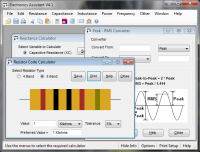# Reactance Calculator

The calculators below find the reactance of capacitors and inductors at a specific AC frequency.

#### Inductive Reactance Calculator (XL = 2pfL):

 Enter Inductance: Henrys Millihenrys Microhenrys Enter Frequency: Hertz Kilohertz Megahertz XL = ?

#### Formulae:

Capacitive reactance:

XC = 1 / (2 * pi * f * C), where f is the frequency and C is the capacitance in Farads

Inductive reactance:

XL = 2 * pi * f * L, where L is the inductance in Henrys

See the technical data section for more details

# If you found this calulator useful you should try Electronics Assistant!• All the online calculators and more in a stand-alone application
• Converts Resistor & Inductor colour codes, calculates LED series resistors, capacitance units, series / parallel resistors & capacitors, frequency, reactance & more
• Calculation of nearest preferred resistor values with a choice of 5 series from E12 to E192
• Print & save calculation results